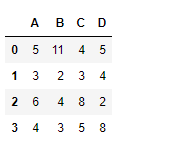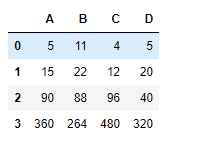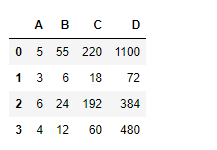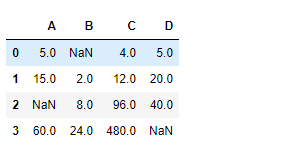Python | Pandas dataframe.cumprod()

• Last Updated : 16 Nov, 2018

Python is a great language for doing data analysis, primarily because of the fantastic ecosystem of data-centric python packages. Pandas is one of those packages and makes importing and analyzing data much easier.

Pandas dataframe.cumprod() is used to find the cumulative product of the values seen so far over any axis.
Each cell is populated with the cumulative product of the values seen so far.

Syntax: DataFrame.cumprod(axis=None, skipna=True, *args, **kwargs)

Parameters:
axis : {index (0), columns (1)}
skipna : Exclude NA/null values. If an entire row/column is NA, the result will be NA

Returns: cumprod : Series

Example #1: Use cumprod() function to find the cumulative product of the values seen so far along the index axis.

 # importing pandas as pdimport pandas as pd  # Creating the dataframedf = pd.DataFrame({"A":[5, 3, 6, 4],                   "B":[11, 2, 4, 3],                   "C":[4, 3, 8, 5],                    "D":[5, 4, 2, 8]})  # Print the dataframedf

Output :Now find the cumulative product of the values seen so far over the index axis

 # To find the cumulative proddf.cumprod(axis = 0)

Output :Example #2: Use cumprod() function to find the cumulative product of the values seen so far along the column axis.

 # importing pandas as pdimport pandas as pd  # Creating the dataframedf = pd.DataFrame({"A":[5, 3, 6, 4],                    "B":[11, 2, 4, 3],                   "C":[4, 3, 8, 5],                    "D":[5, 4, 2, 8]})  # cumulative product along column axisdf.cumprod(axis = 1)

Output :Example #3: Use cumprod() function to find the cumulative product of the values seen so far along the index axis in a data frame with NaN value present in dataframe.

 # importing pandas as pdimport pandas as pd  # Creating the dataframedf = pd.DataFrame({"A":[5, 3, None, 4],                   "B":[None, 2, 4, 3],                    "C":[4, 3, 8, 5],                    "D":[5, 4, 2, None]})  # To find the cumulative productdf.cumprod(axis = 0, skipna = True)

Output :The output is a dataframe with cells containing the cumulative product of the values seen so far along the index axis. Any Nan value in the dataframe is skipped.

My Personal Notes arrow_drop_up